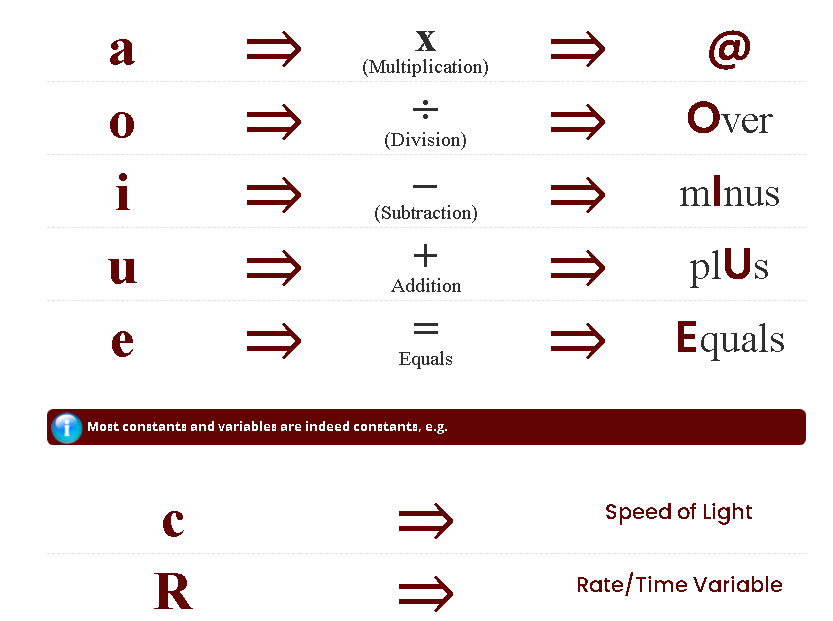## Mnemonic Writing

While in the Army, Mr. Schunicht was involved in a mid-air collision rendering him unconscious for three weeks, everything had to be relearned as nursing actions are reported as having been displayed upon awakening from the extended unconsciousness (19 days).

Studies in recovery brought about some pragmatic discoveries to compensate for the residual memory deficits. The most valuable discovery was having each vowel represent a mathematical sign, i.e.

## MATHEMATICAL SIGNS REPRESENTED BY VOWELS## EQUATIONS TO ACRONYMS

Here is a couple of examples I can walk you through on how to take a simple equation and make it even easier to remember!

WAVELENGTH

#### Step 1

Wavelength step one consists of the following symbols. Using these symbols to represent the equation makes it extremely simple to memorize.λ = wavelength
λC= h/(mec) Compton wavelength
c = speed of light,
c = 2.426310238(16) x 10-12m
γ = frequency (nu)

#### Step 2

Wavelength step one consists of the following symbols. Using these symbols to represent the equation makes it extremely simple to memorize.λ = wavelength
c = speed of light,
γ = frequency (nu)

#### Step 3

Wavelength step one consists of the following symbols. Using these symbols to represent the equation makes it extremely simple to memorizeλ = wavelength
c = speed of light,
γ = frequency (nu)

#### Step 4

Wavelength step one consists of the following symbols. Using these symbols to represent the equation makes it extremely simple to memorize.λ = wavelength
c = speed of light,
γ = frequency (nu)